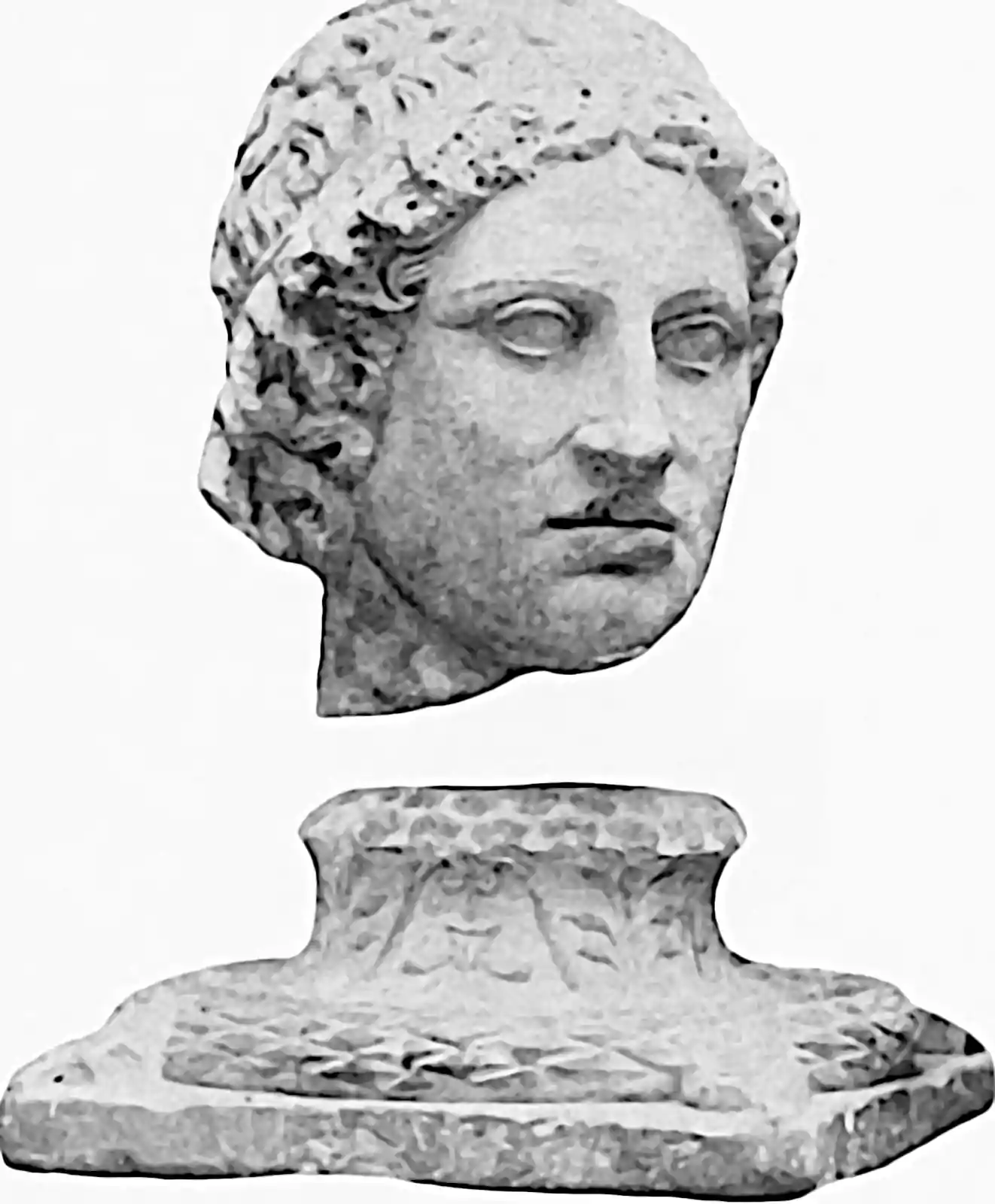# Generalised Ornstein-Uhlenbeck processes

## Markov/AR(1)-like processes

$\renewcommand{\var}{\operatorname{Var}} \renewcommand{\corr}{\operatorname{Corr}} \renewcommand{\dd}{\mathrm{d}} \renewcommand{\bb}{\mathbb{#1}} \renewcommand{\vv}{\boldsymbol{#1}} \renewcommand{\rv}{\mathsf{#1}} \renewcommand{\vrv}{\vv{\rv{#1}}} \renewcommand{\disteq}{\stackrel{d}{=}} \renewcommand{\gvn}{\mid} \renewcommand{\Ex}{\mathbb{E}} \renewcommand{\Pr}{\mathbb{P}}$

Ornstein-Uhlenbeck-type autoregressive, stationary stochastic processes, e.g. stationary gamma processes, classic Gaussian noise Ornstein-Uhlenbeck processes… There is a family of such induced by every Lévy process via its bridge.## Classic Gaussian

### Discrete time

Given a $$K \times K$$ real matrix $$\Phi$$ with all the eignevalues of $$\Phi$$ in to the interval $$(-1,1)$$, and given a sequence $$\varepsilon_t$$ of multivariate normal variables $$\varepsilon_t \sim \mathrm{N}(0, \Sigma)$$, with $$\boldsymbol{\Sigma}$$ a $$K \times K$$ positive definite symmetric real matrix, the stationary distribution of the process $\mathbf{x}_t=\varepsilon_t+\boldsymbol{\Phi} \mathbf{x}_{t-1}=\sum_{h=0}^t \boldsymbol{\Phi}^h \varepsilon_{t-h} \quad ?$ is given by the Lyapunov equation, or just by basic variance identities. It is Gaussian with $$\mathcal{N}(0, \Lambda)$$ where the following recurrence relation holds for $$\Lambda$$, $\Lambda=\Phi \mathbf{x} \Phi^{\top}+\Sigma.$ The solution is also, apparently, the limit of a summation $\Lambda=\sum_{k=0}^{\infty} \Phi^k \Sigma\left(\Phi^{\top}\right)^k.$

### Continuous time

Suppose we use a Wiener process $$W$$ as the driving noise in continuous time with some small increment $$\epsilon$$, $d \mathbf{x}(t)=-\epsilon A \mathbf{x}(t) d t+ \epsilon B d W(t)$ This is the Ornstein-Uhlenbeck process. If stable, at stationarity it an analytic stationary density $$\mathbf{x}\sim\mathcal{N}(0, \Lambda)$$ where $\Lambda A+A \Lambda =\epsilon B B^{\top}.$

## Gamma

Over at Gamma processes, Wolpert (2021) notes several example constructions which “look like” Ornstein-Uhlenbeck processes, in that they are stationary-autoregressive, but constructed by different means. Should we look at processes like those here?

For fixed $$\alpha, \beta>0$$ these notes present six different stationary time series, each with Gamma $$X_{t} \sim \operatorname{Ga}(\alpha, \beta)$$ univariate marginal distributions and autocorrelation function $$\rho^{|s-t|}$$ for $$X_{s}, X_{t} .$$ Each will be defined on some time index set $$\mathcal{T}$$, either $$\mathcal{T}=\mathbb{Z}$$ or $$\mathcal{T}=\mathbb{R}$$

Five of the six constructions can be applied to other Infinitely Divisible (ID) distributions as well, both continuous ones (normal, $$\alpha$$-stable, etc.) and discrete (Poisson, negative binomial, etc). For specifically the Poisson and Gaussian distributions, all but one of them (the Markov change-point construction) coincide— essentially, there is just one “AR(1)-like” Gaussian process (namely, the $$\operatorname{AR}(1)$$ process in discrete time, or the Ornstein-Uhlenbeck process in continuous time), and there is just one $$\operatorname{AR}(1)$$-like Poisson process. For other ID distributions, however, and in particular for the Gamma, each of these constructions yields a process with the same univariate marginal distributions and the same autocorrelation but with different joint distributions at three or more times.

### No comments yet. Why not leave one?

GitHub-flavored Markdown & a sane subset of HTML is supported.